# Curve Drawing toolbar

#####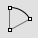Arc

Draw an arc with options for center, start, angle, and direction.

Link to Arc toolbar

#####Arc, Start, End, Direction

Arc by start, end, direction at start.

#####Arc, Start, Direction, End

Arc by start, direction at start, end.

#####Arc, Start, End, Point

Arc by start, end, point on arc.

#####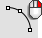Arc, Start, Point, End

Arc by start, point on arc, end.

#####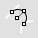Arc, Tangent

Arc tangent to curves.

#####Arc, Tangent, Tangent, Radius

Arc tangent, tangent, radius.

#####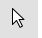Cancel

Cancel the current command and deselects objects.

#####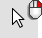Cancel, All

Turns off Points, CurvatureGraph, EMap, Zebra, MoveUVN, CurvatureAnalysis, and deselects objects.

#####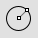Circle

Draw a circle from center and radius, diameter, points on the circumference, and circumference length.

Link to Circle toolbar

#####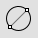Circle, Diameter

Draws a circle from points on diameter.

#####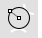Circle, Tangent, Tangent, Radius

Draws a circle tangent to two curves and a radius.

#####Circle, Vertical to CPlane: center, radius

Draws a circle from a center and radius vertical to construction plane.

#####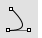Conic, Tangent at start

Draw a conic curve tangent at the start.

#####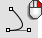Conic, Tangent at start and end

Draw a conic curve tangent at the start and end.

#####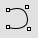Curve

Draw a curve from control point locations.

Link to Curve toolbar

#####CurveThroughPt

Fit a curve through point objects.

#####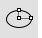Ellipse

Draw a closed elliptical curve from focus points, center and edges, bounding rectangle, and around a curve.

Link to Ellipse toolbar

#####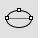Ellipse, Diameter

Draws a closed elliptical curve by diameter.

#####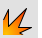Explode

Break objects down into components.

#####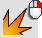ExtractSrf

Separate or duplicate a polysurface face.

#####HandleCurve

Draw chained Bézier curves with editing handles.

#####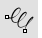Helix

Draw a helical curve with options for number of turns, pitch, vertical, reverse, and around a curve.

#####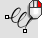Helix, Vertical

Draw a vertical helical curve.

#####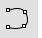InterpCrv

Fit a curve through picked locations.

#####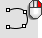HandleCurve

Draw chained Bézier curves with editing handles.

#####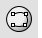InterpCrvOnSrf

Fit a curve through locations on a surface.

#####Join

Connect curves, surface edges, or surfaces to form a single object.

#####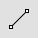Line

Draw a single line segment.

#####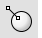Line, Normal

Draw a line segment normal to a surface.

#####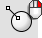Line, Normal, BothSides

Draw a line segment normal to a surface from its midpoint.

#####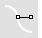Line, Perpendicular, from curve

Draw a line segment perpendicular from a curve.

#####Line, Perpendicular, to curve

Draw a line segment perpendicular to a curve.

#####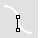Line, Tangent

Draw a line segment tangent from a curve.

#####Line, Vertical

Draw a line segment perpendicular to the construction plane.

#####Line, Vertical, BothSides

Draw a line segment perpendicular to the construction plane from its midpoint.

#####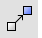Move

Move objects from one location to another.

Link to Transform toolbar

#####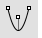Parabola

Draw a parabolic curve from focus and vertex or endpoint.

#####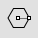Polygon

Draw a polygon with a specified number of sides with options for inscribed/circumscribed, by edge, star-shaped, around a curve, and vertical.

Link to Polygon toolbar

#####Polygon, Square from center and corner

Draw a square polygon by center and corner.

#####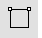Polygon, Square, from edge

Draw a square polygon by edge.

#####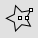Polygon, Star

Draw a polygon star.

#####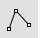Polyline

Draw a multi-segment polyline with options for line and arc segments, tracking line helpers, and close.

Link to Lines toolbar

#####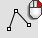Lines

Draw multiple adjoining line segments.

#####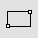Rectangle

Draw a rectangular closed polyline from with options for starting at the center, three points, vertical, and rounded with arc or conic corners.

Link to Rectangle toolbar

#####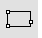Rectangle, 3Point

Draw a rectangle through three points.

#####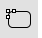Rectangle, Rounded

Draw a rectangle with rounded corners.

#####Rectangle, Rounded, conic corners

Draw a rectangle with conic rounded corners.

#####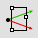Rectangle, Vertical

Draw a rectangle perpendicular to the construction plane.

#####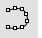Sketch

Drag the mouse to draw a curve.

#####Sketch, on surface

Drag the mouse to draw a curve on a selected surface.

#####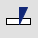Split

Divide objects using other objects as cutters.

#####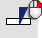Split, Isocurve

Divide objects using isocurves as cutters.

#####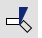Trim

Cut and delete selected portions of an object at the intersection with another object.

#####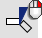Untrim

Remove trim curves from trimmed surfaces.

Rhino for Mac © 2010-2017 Robert McNeel & Associates. 24-Oct-2017